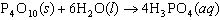# Stoichiometry Practice Test

Download 263.82 Kb.
 Date 20.02.2019 Size 263.82 Kb.
 Stoichiometry Practice Test ____ 1. How many moles of silver atoms are in 1.8 10 atoms of silver? a. 3.0 10 b. 3.3 10 c. 3.0 10 d. 1.1 10 ____ 2. How many atoms are in 0.075 mol of titanium? a. 1.2 10-25 b. 2.2 10 c. 6.4 10 d. 4.5 10 ____ 3. What is true about the molar mass of chlorine gas? a. The molar mass is 35.5 g. b. The molar mass is 71.0 g. c. The molar mass is equal to the mass of one mole of chlorine atoms. d. none of the above ____ 4. What is the molar mass of AuCl3? a. 96 g b. 130 g c. 232.5 g d. 303.6 g ____ 5. What is the molar mass of (NH)CO? a. 144 g b. 138 g c. 96 g d. 78 g ____ 6. In a chemical reaction, the mass of the products ____. a. is less than the mass of the reactants b. is greater than the mass of the reactants c. is equal to the mass of the reactants d. has no relationship to the mass of the reactants ____ 7. In any chemical reaction, the quantities that are preserved are ____. a. the number of moles and the volumes b. the number of molecules and the volumes c. mass and number of atoms d. mass and moles ____ 8. How many moles of HPO are produced when 71.0 g PO reacts completely to form HPO?a. 0.063 5 mol b. 1.00 mol c. 4.00 mol d. 16.0 mol ____ 9. How many grams of HPO are produced when 10.0 moles of water react with an excess of PO?a. 1.22 g b. 6.7 g c. 147 g d. 653 g 10. Find the mass in grams of 3.10 10 molecules of F. 11. Solid sodium reacts violently with water, producing heat, hydrogen gas, and sodium hydroxide. How many molecules of hydrogen gas are formed when 48.7 g of sodium are added to water? 2Na + 2HO 2NaOH + H 12. In your stoich calculations, what is the importance of the coefficients in a balanced chemical reaction? Stoichiometry Practice Test Answer Section MULTIPLE CHOICE 1. ANS: A REF: p. 290 | p. 291 2. ANS: D REF: p. 291 | p. 292 3. ANS: B REF: p. 294 4. ANS: D REF: p. 295 | p. 296 5. ANS: C REF: p. 295 | p. 296 6. ANS: C REF: p. 356 7. ANS: C REF: p. 356 8. ANS: B REF: p. 360 | p. 361 | p. 362 9. ANS: D REF: p. 360 | p. 361 | p. 362 SHORT ANSWER 10. ANS: 3.10 10 molecules 1 mol F/6.02 10 molecules 38.0 g F/1 mol F = 19.6 g F REF: p. 297 11. ANS: Assume the sodium is limiting: 48.7 g Na 1 mol Na/23.0 g Na 1 mol H/2 mol Na (6.02 10 molecules H)/1 mol H = 6.37 10 molecules H REF: p. 371 ESSAY 12. ANS: The coefficients in a balanced chemical equation indicate the relative number of moles of reactants and products. From this information, the amounts of reactants and products can be calculated. The number of moles may be converted to mass, volume, or number of representative particles. REF: p. 356Download 263.82 Kb.Share with your friends:

The database is protected by copyright ©sckool.org 2020
send message

Main page